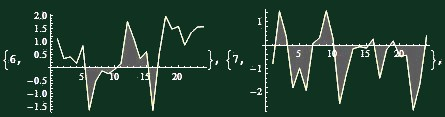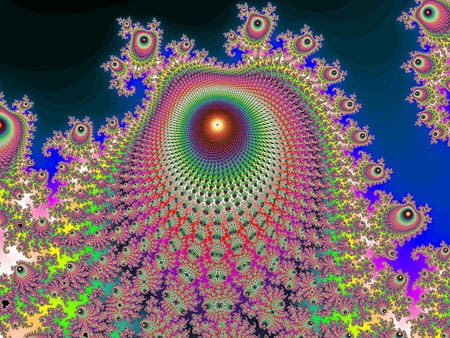Wednesday, March 24, 2010

Self-similarity of temperature graphs

Tamino, virtually all champions of the climate panic, and even most skeptics tend to think that the fluctuations and noise of the temperature graphs only exist at the short time scales.

They think that when we compute the average over sufficiently long timescales, i.e. many years or a few decades, the signal becomes bigger than the noise and the trends show up. For example, they think that if you look at more than 15 years, the warming trend becomes visible and very different from the noise.

However, this assumption is completely wrong.

The graphs of temperature anomalies - between weeks and millennia - actually look like some kind of pink noise. Much like red noise, the pink noise has the property that if you focus on a part of it and you scale the picture to fill the whole screen, both in the horizontal (time) and vertical (temperature) direction (these two scale factors may differ), you always get the same qualitative picture. The signal-to-noise ratio is actually pretty much independent of the time scale you choose - a typical behavior that people know from "critical phenomena" or "conformal field theories".

Using a simple adjective, the graphs are self-similar.Here is my homework problem that will explain you what I mean.

Click the picture above (and then the thumbnail, twice) and you will get 100 similar graphs on a huge image (that won't fit your screen, but you can scroll).

Each graph contains 24 datapoints from Central England. First, I calculated the temperature anomaly for the 4,214 known months (from January 1659 to February 2010): the anomaly is the difference of the average temperature for a month minus the average temperature for all months with the same name from the whole 1659-2010 interval.

Then I randomly generated 100 pairs of the following two numbers:
• resolution - which may be either 1,2,4,8,16,32,64, or 128 months
• first month - a random month between 1659 and 2010 so that the whole graph can be drawn with the known data
With these numbers, I drew 100 graphs consisting of 24 datapoints each. The datapoints show the average temperature in 24 consecutive intervals. The length of each of these intervals is given by the resolution. Because 24 months is equal to two years, the total period of time captured by each graph is twice the resolution in years, so it is
• 2,4,8,16,32,64,128, or 256 years
These are vastly different timescales. The longest one is 128 times longer than the shortest one. The temperature should look very different for each - but it doesn't. By the way, I divided the description of the y-axis by a power of the resolution so that you can't quite see the overall normalization of the temperature changes in each graph.

Now, your task is to open the image with the 100 homework problems and tell me - at least approximately - what is the time resolution of each of them.

My guess is that you will fail completely. For example, you can choose logarithmic symbols 0,1,2,3,4,5,6,7 for the time resolutions above.

When you're finished, you should look at the solution, another huge JPG image. In the solution, each graph not only has the ID - between 1 and 100 - but also the information about the beginning of the graph on the left and the resolution.

Take the base-two logarithm of the correct resolutions - and subtract them from your "logarithmic symbols". Sum up the absolute values. If you were guessing 3.5 (or 3 or 4) for all graphs, your total deviation from the 100 graphs would be (exactly, in all cases) 200. Check whether you can get much closer to the truth than 200: if you could guess the resolution, your total deviation would be 0. I actually guess that your total error will even be bigger than 200 - bigger than what you get by an algorithm in which you don't look at the pictures at all while guessing. ;-)For example, the two graphs whose ID were 6 and 7 that I originally cropped captured a 2-year period around 1958 (left) and a 64-year period started around 1756 (right). Still, there is really no qualitative difference between them. In fact, the "trend" (or a trend-like increase) over the two years on the left graph seems bigger than the "trend" over the 64 years on the right graph.

Be sure that this is not because the climate changes have sped up 32 times, between 1756 and 1958. They surely haven't. ;-) It's because at each timescale, the persistence of the temperature - and therefore the "signal-to-noise ratio" - remains largely constant. Frankly speaking, the graphs would qualitatively look almost identical even if you covered a 24,000-year period, with the 24 individual points describing average temperatures over 1,000 years!Much like random walks, fractals exhibit self-similarity.

The lessons, repeated

The main lesson is that the graphs are never quite "smooth" or even "linear" but they're never quite "white noise", either. (White noise is completely discontinuous.) The "color" or "relative degree of persistence" is actually pretty much independent of the time scale.

The atmosphere turns out to be affected by chaotic - or de facto unpredictable - influences "almost equally" at all time scales. This proposition actually holds between days and millennia. Only when the time scales are comparable to 10,000 years or longer, the typical separation between ice ages and interglacials, the climate starts to get "stabilized" so that the temperature changes no longer grow with the separation and are kept within a 10 °C window or so.

(10,000 years is the scale above which the self-similarity starts to disappear; people's intuition usually suggests that the timescale should be much shorter - but the causes behind this intuition are irrational. The Earth is big and its natural dynamics is linked to long distance scales as well as long time scales.)

But the fluctuations at shorter time scales than that - any time scales that are relevant for the planning of the human society - always exist and always increase with the time separation - but parametrically more slowly than the linear growth. That's how the natural variations and cycles work.

You should understand that a linear growth would predict that the temperature change "delta T" is proportional to the time "t". Clearly, the climate never behaves in this way. The white noise predicts that the temperature change "delta T" is independent of the time separation "t": the deviations from the "normal" would be independent (uncorrelated) at any two different moments (a constant is proportional to the zeroth power of "t" - i.e. to one). Clearly, this is not true, either.

A good model for the reality is that the temperature change after time "t" is close to a power of "t", namely "t^k", where the exponent "k" is between 0 and 1. Red noise - the Brownian motion - would imply "k=1/2". The real world leads to different values of "k" between zero and one. But the qualitative intuition from the Brownian motion (or random walk) is fully applicable.

Whatever the value of "k" is, it is true that the graphs are slightly continuous - i.e. they contain "trends" that are actually random flukes as well - at each time scale. It would always be a mistake to extrapolate these trends because they're noise - just a different kind of noise than the laymen are usually familiar with (which is "white noise").

One more comment. Some people might think that only random walk, i.e. noise with the "k=1/2" exponent, can appear in Nature. (Red noise or random walk can be easily obtained as the integral of white noise.) However, that's not the case. Any fractional exponent may emerge in Nature. It is actually a rule rather than the exception that random fractional exponents "k" govern the critical behavior of complex enough systems similar to the climate (or something very different).

One implication of these facts is that if you can get a (modestly) statistically significant trend by looking at 16 years - their annual mean temperatures - it doesn't mean that this statistical signifance proves that the "trend" is anything else than noise. Such "trends" are actually omnipresent at all time scales and virtually all of them that you can see in my homework are noise - and their extrapolation has always failed.

You get the same "apparent trend" which is equally statistically significant (i.e. it is demonstrably - at a moderately suggestive confidence level - not white noise) if you look e.g. at a pretty generic set of 16 consecutive monthly anomalies, too! If we were looking at the global mean temperature rather than Central England, the short-term graphs would actually look even more continuous and "having a trend" than the English data!

For shorter time scales, the "trend" would be many or dozens of degrees of per century. Such trends look as "real" as the trends observed at graphs over 30 or 100 years. A sufficient number of points - e.g. 16 - can produce "statistically significant" trends.

But they're actually noise: the only thing that the conventionally calculated "statistical significance" proves that they're not white noise. Indeed, the temperature graphs are never white noise. There is no reason to think that the situation is any different for the 16-year or 30-year "trends". Such "trends" are always spurious: they're part of the noise produced by Nature and it is a misconception to interpret them as some "signals".

And that's the memo.

P.S.: You may download the Central England Temperature Mathematica 7 notebook that was used to generate the 100 graphs.

1.2.